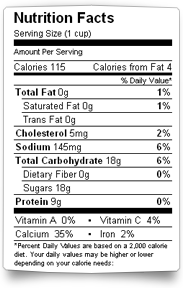Learn the daily calories needed to achieve your fitness goals. Your calorie requirements should be determined by your genetics, weight, activity level and fitness goals. There is a minimal caloric need for your body to function properly. Calorie needs is also slightly different for males and females.

A larger individual will generally require more calories than a smaller individual.  A 150 pound male may only require 1400 calories a day while a 250 pound guy may require 1800 per day just to maintain enough energy to get through the day. The activity level of a person also determines how many daily calories are required. A female the exercises 5 days a week and jogs will require more energy than a person that lives a sedentary lifestyle. Your fitness goals also are a determinate in your calorie needs. If you are trying to lose weight, you may want to lower your calorie intake. If a male is trying to pack on muscle mass, he may decide to increase his calorie intake. You must be careful not to go to low because this can have a negative effect on your body functions.

## Harris Benedict Calculator

Below is a Harris-Benedict Calculator (BMR calculator) that computes your Basal Metabolic Rate (the amount of calories you would burn if you were asleep all day). This calculator gives you results based on the Harris-Benedict formula (equation)

Just enter your data below and click the “calculate” button.

Document

# Calculate Basal Metabolic Rate (BMR) online (Harris Benedict Equation)

Your Basal Metabolic Rate (BMR is the amount of calories you would burn if you were asleep all day. The Harris Benedict Equation determines your total daily energy expenditure (calories).

## Your BMR

• BMR:
• 448
• Daily Calorie Needs:
• 627 calories

## Calorie Requirements for Men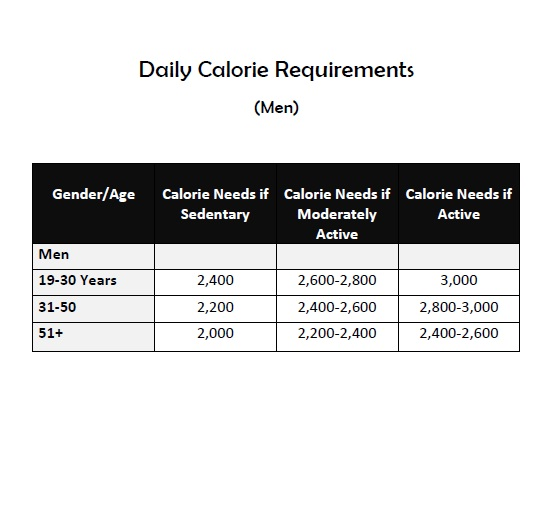Below is a calorie requirements chart for men. This chart will give you a rough estimate of the amounts of calories you should intake daily. You may download and print for your convience.

## Calorie Requirements for Women

This printable chart will give you a rough estimate of the amounts of calories you should intake daily. You may download and print for your convenience.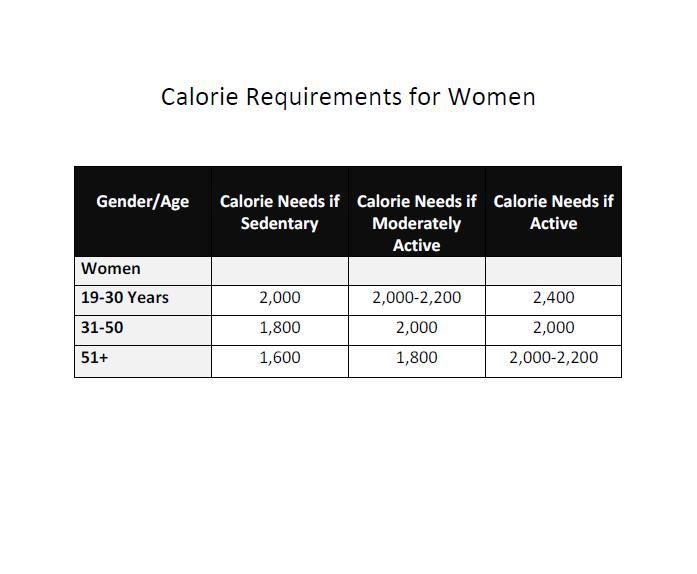Gender/Age Calorie Needs if Sedentary Calorie Needs if Moderately Active Calorie Needs if Active Women 19-30 Years 2,000 2,000-2,200 2,400 31-50 1,800 2,000 2,000 51+ 1,600 1,800 2,000-2,200

## Eating to Gain Muscle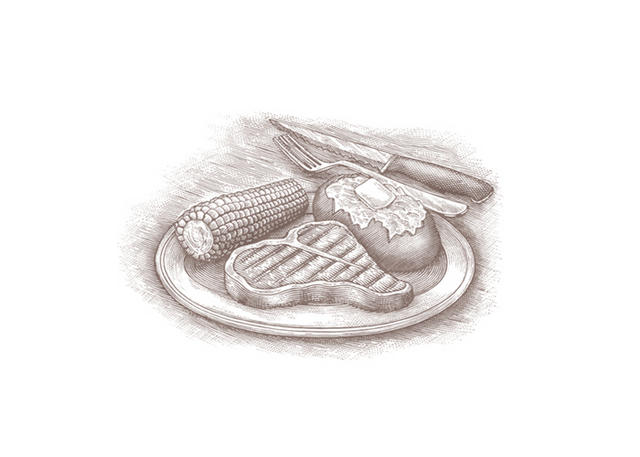If your primary goal is to gain muscle, eating right is a key component. Here are 6 tips for healthful weight gain.

## Calorie Needs Calculator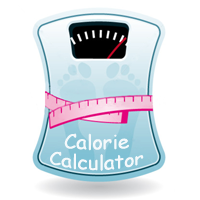Knowing how many calories you should consume varies with each individual. You must feed your body enough calories for it to function properly. Factors such as weight, height, gender and activity level are used to determine your calorie needs. Here's a handy tool below to give you a rough calorie needs estimate.

## Calculating your Resting Energy Expenditure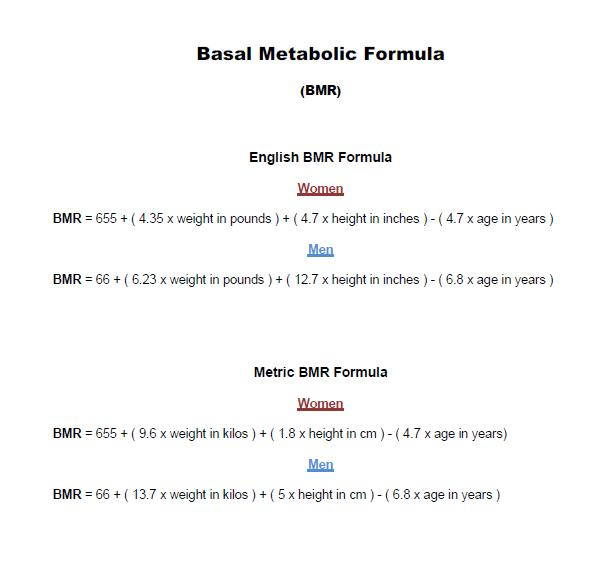The Harris Benedict Equation is a formula that uses your BMR (Resting Energy Expenditure) and then applies an activity factor to determine your total daily energy expenditure (calories). The only factor omitted by the Harris Benedict Equation is lean body mass. Remember, leaner bodies need more calories than less lean ones. Therefore, this equation will be very accurate in all but the very muscular (will under-estimate calorie needs) and the very obese (will over-estimate calorie needs).

## How many calories do you need?

To get a rough estimate of how many calories you need in a day to maintain your present weight, multiply your weight by 13, if sedentary, and by 15, if moderately active. You need to add more calories for rigorous exercise.

To lose or gain weight, subtract or add 500 calories through diet and exercise. For example to lose about a pound a week, decrease your calorie intake by 250 calories and increase the calories you burn off through exercise by 250. Walking one mile briskly in 15 minutes burn approximately 100 calories.

## Harris Benedict Formula Example

Here's a Harris Benedict Formula example of how to determine the amount of calories (BMR rate) you burn while resting.

WARNING: Use this equation only as an estimate.

Example 1

Susan is 45 years old; she weighs 245 pounds and is 5 foot 5 inches tall. She works a job where she sits most of the day. She never exercises. Based on these statistics, how many calories is Susan daily?

Step 1

Plug Susan's weight (245lbs), height (5 foot 5 inches), and age (45 yrs) into the formula below for women to find her BMR.

 English BMR Formula Women: BMR = 655 + (4.35 x weight in pounds) + (4.7 x height in inches) - (4.7 x age in years)Men: BMR = 66 + (6.23 x weight in pounds) + (12.7 x height in inches) - (6.8 x age in year)

BMR=655 + (4.35 x weight in pounds) + (4.7 x height in inches) - (4.7 x age in   years).

BMR=655 + (4.35 x 245)  + (4.7 x 65) - (4.7 x 45)

BMR=655 + 1065.75 + 305.5 - 211.5

BMR= 1814.75

Step 2

Determine Susan's activity level using the chart below

 Harris Benedict Formula To determine your total daily calorie needs, multiply your BMR by the appropriate activity factor, as follows:   *  If you are sedentary (little or no exercise): Calorie-Calculation = BMR x 1.2   *  If you are lightly active (light exercise/sports 1-3 days/week): Calorie-Calculation = BMR x 1.375   *  If you are moderately active (moderate exercise/sports 3-5 days/week): Calorie-Calculation = BMR x  1.55   * If you are very active (hard exercise/sports 6-7 days a week): Calorie-Calculation = BMR x 1.725   *  If you are extra active (very hard exercise/sports & physical job or 2x training): Calorie-Calculation = BMR x 1.9

Step 3

Plug numbers into the formula

Since Susan doesn't exercise and inactive she is sedentary according the chart above. Based on that information, multiply her BMR X 1.2

Plug in the appropriate numbers

Susan's calories burned while at rest = SUSAN'S BMR X 1.2

Susan's calories burned while at rest = 1814.75 x 1.2 = 2177.7

Susan burns approximately 2177.7 calories a day at rest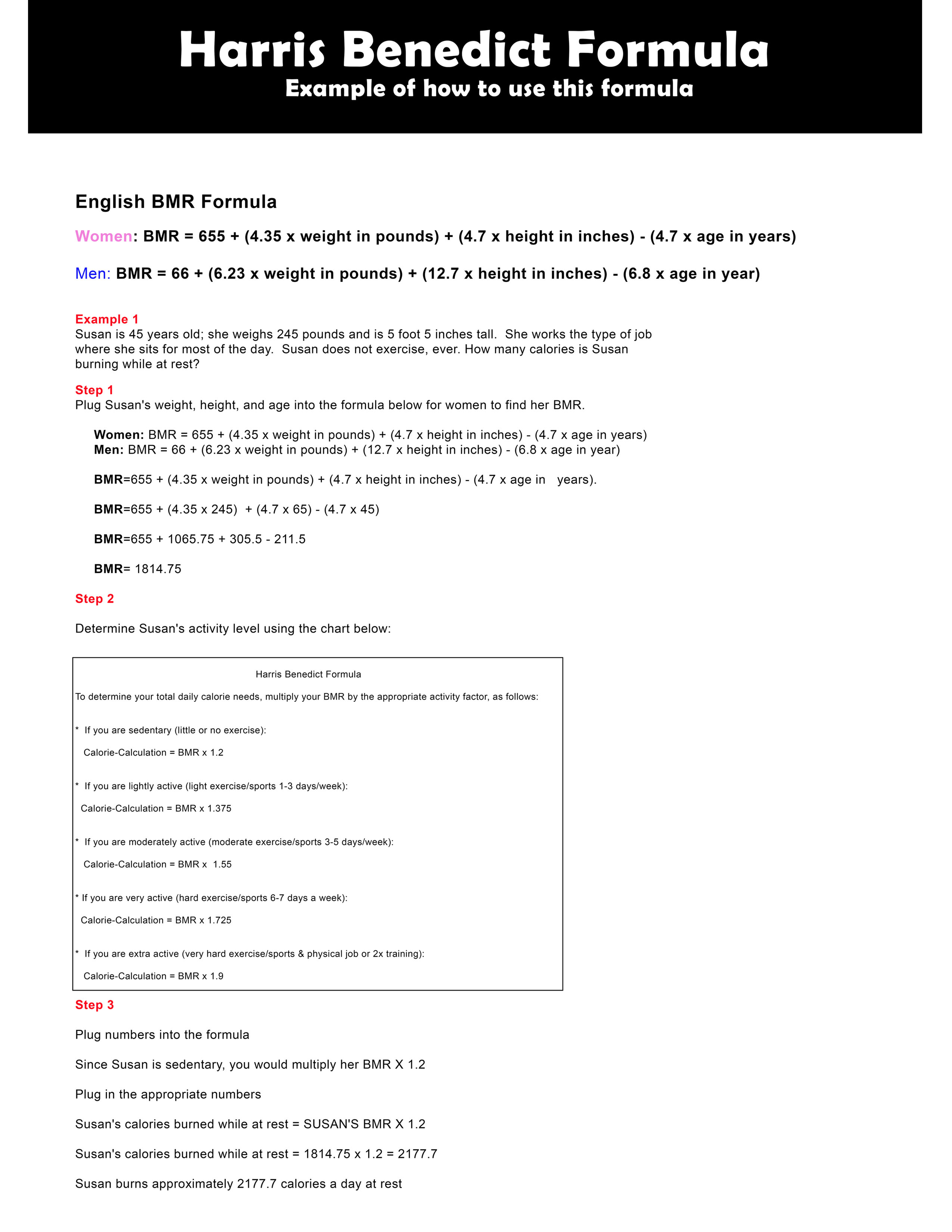Download Medium size (17" by 11") Harris Benedict formula chart.